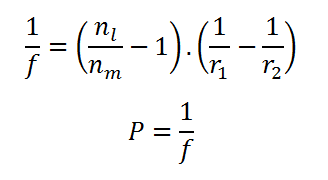# Lensmaker Equation Calculator

This CalcTown calculator calculates the focal length of a bifocal lens using Lensmaker's formula.

CARTESIAN CONVENTION:

1) Light initially propagates from left to right.

2) The origin of the Cartesian coordinate system is at the centre of the optical component.

3) Distances measured normal to the optic axis are positive above and negative below.

4) We denote object space distances as l, h, f, and image space distances as l ', h', f '.

5) Acute angles are positive when produced by anticlockwise rotation from the optic axis, and negative when produced by clockwise rotation.

#### Result

cm
Diopter